# Hoisting(变量提升)

``````var x = 1;
console.log(x + " " + y);  // '1 undefined'
catName("Chloe")        //'Choloe'

var y = 2;
function catName(name) {
console.log("我的猫名叫 " + name);
}``````

``````var x=1;
var y;
console.log(x + " " + y);  // '1 undefined'

function catName(name) {
console.log("我的猫名叫 " + name);
}
y = 2;

catName("Chloe")        //'Choloe'``````

``````var username = 'hpdoger';

function echoName(){
}

echoName();``````

# 变量升级

## 声明错误

``````function echoName(){
nickname = 'wuyanzu';
}

function CheckVal(){
console.log(nickname); //wuyanzu
}

echoName();
CheckVal();``````

### 案例1-Fake Protect

``````<html>
<body>
<script>
const whiteList = ['index.html','404.html','hpdoger.html'];

function init(){
const Content = new Object();
Content["title"] = "XSS Demo";
page = location.hash.slice(1);

if(!whiteList.includes(page)){
Content["page"] = "404.html";
}else{
window.page = page;
}
return Content;
}

window.open(page);
}

let Content = init();

</script>
</body>

</html>``````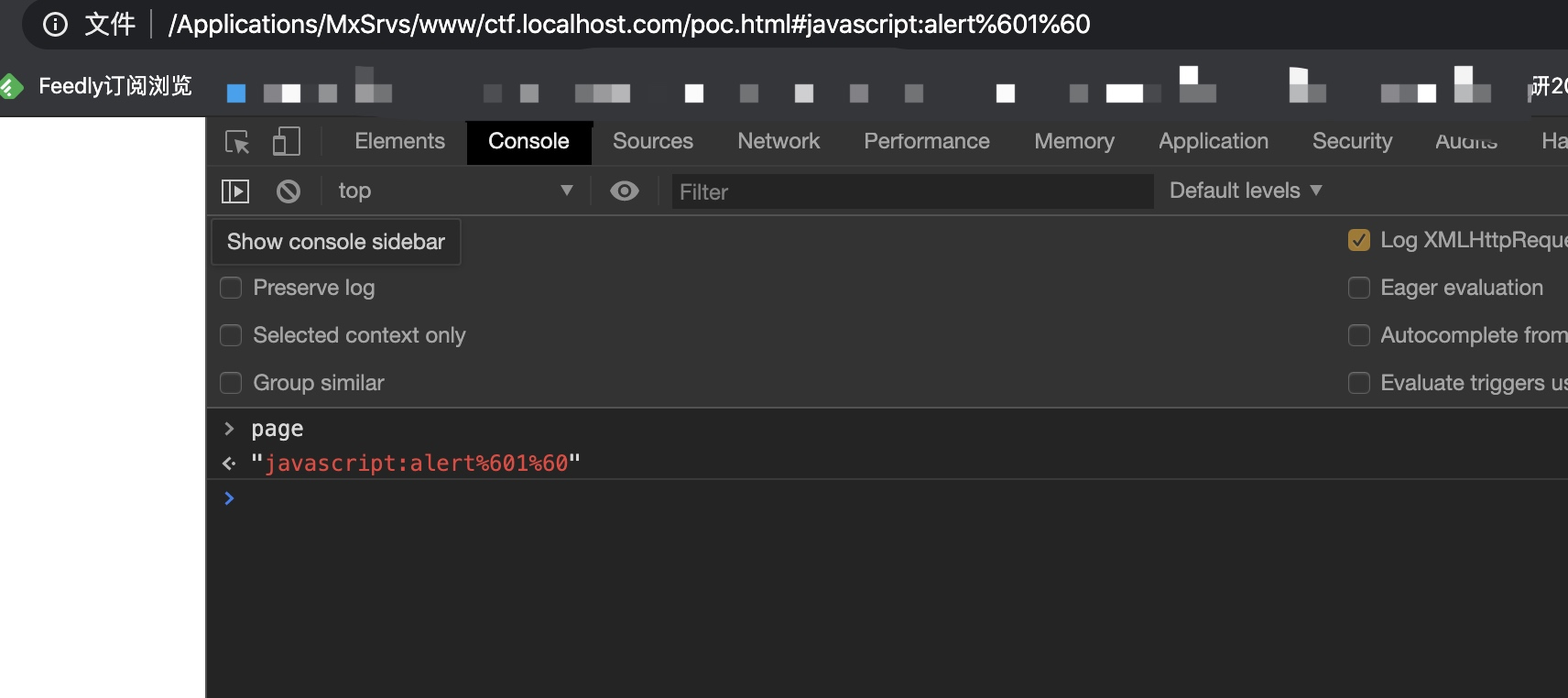### 案例2-midnightCTF(Crossintheroof)

XSS题目，要求我们能够`alert(1)`即可``````<?php

if(!isset(\$_GET['xss'])){
if(isset(\$_GET['error'])){
die('stop haking me!!!');
}

if(isset(\$_GET['source'])){
highlight_file('index.php');
die();
}

die('unluky');
}

\$xss = \$_GET['xss']?\$_GET['xss']:"";
\$xss = preg_replace("|[}/{]|", "", \$xss);

?>
<script>
setTimeout(function(){
try{
return location = '/?i_said_no_xss_4_u_:)';
nodice=<?php echo \$xss; ?>;
}catch(err){
return location = '/?error='+<?php echo \$xss; ?>;
}
},500);
</script>
<script>
/*

*/
</script>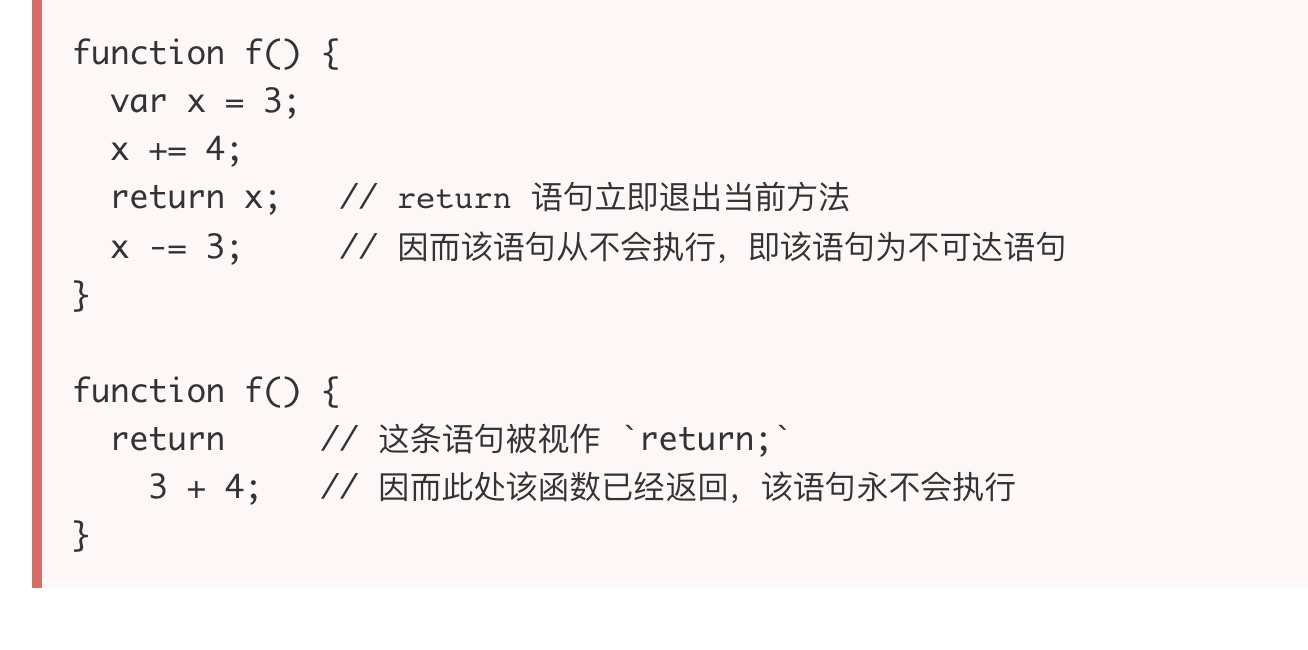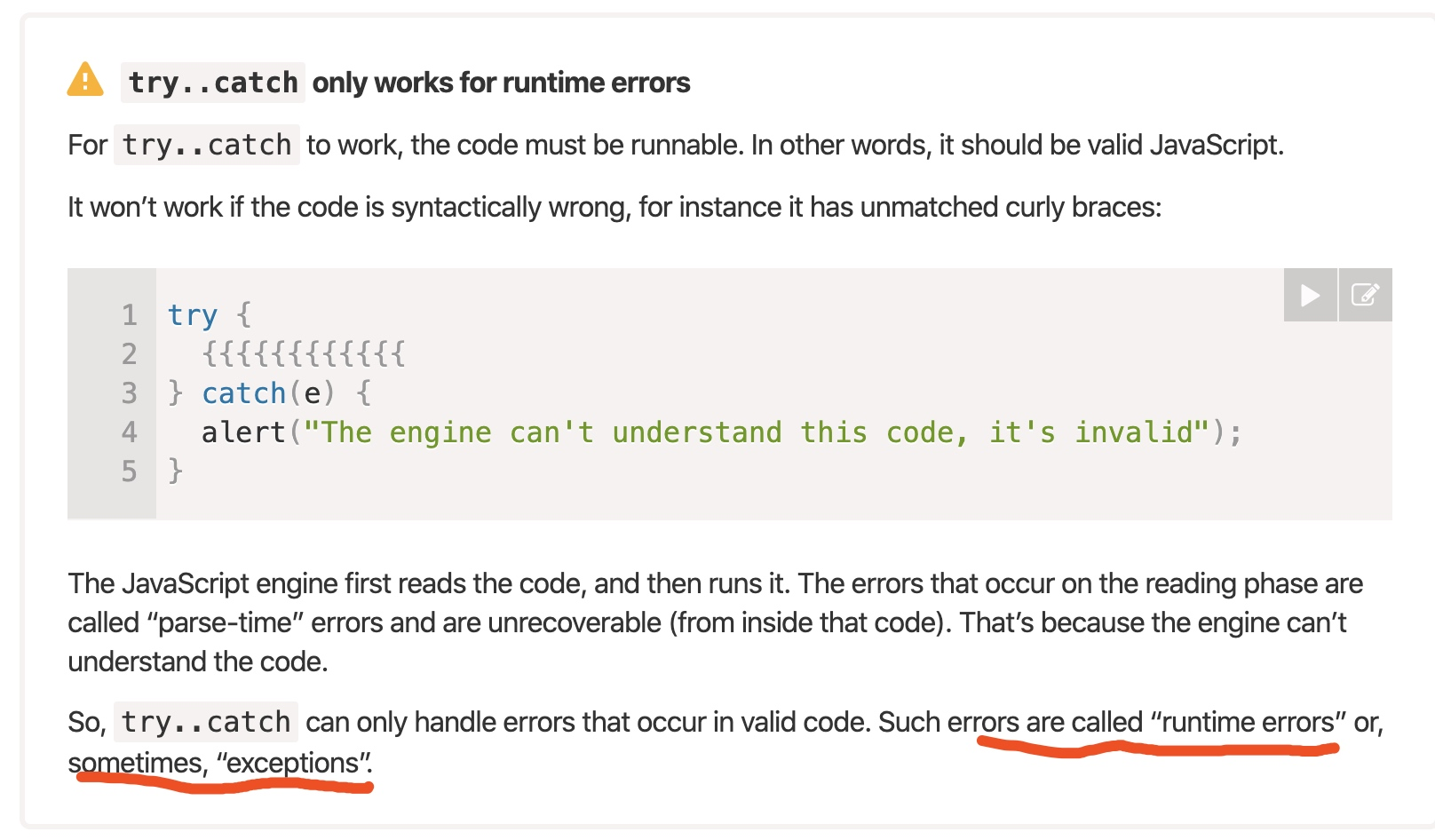``xss=alert(1);%0a+const+location=1;``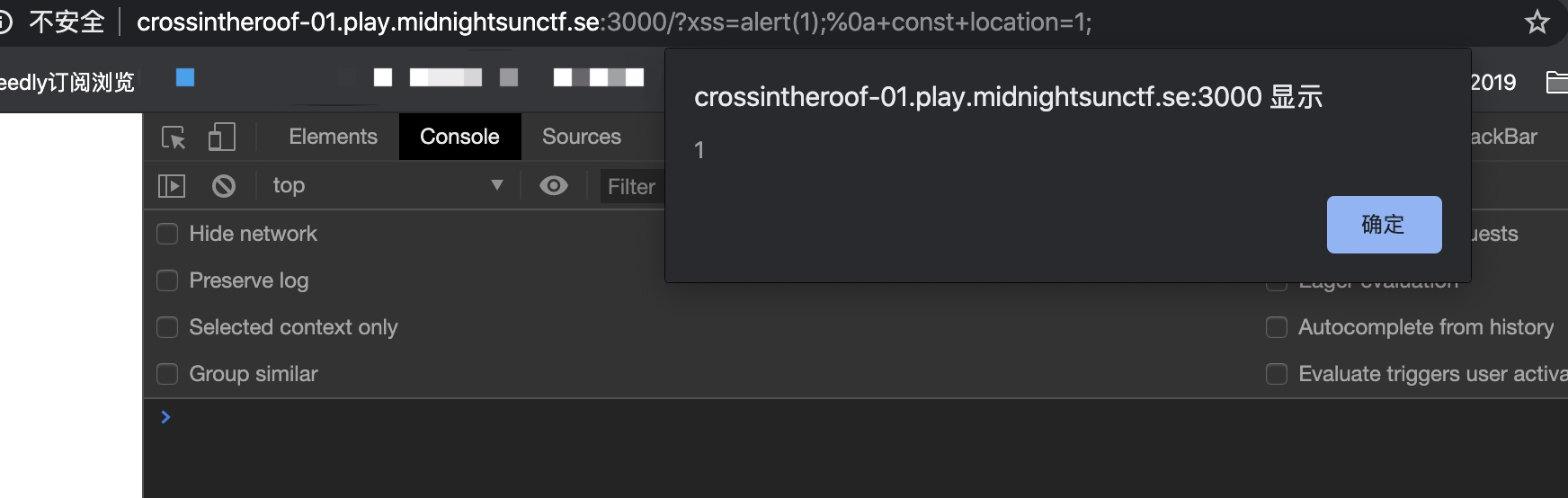## for循环遍历

for中使用var定义变量时也存在升级的问题。这种案例到处都是，我们就仿照菜鸟教程上关于`for`示例的写法来打印一个数组

``````names = ['55kai','pdd','dasima'];
for (var i=0;i<names.length;i++)
{
if(names[i] === 'dasima'){
console.log('wuhu~');
}else{
console.log(names[i]);
}
}``````

## with表达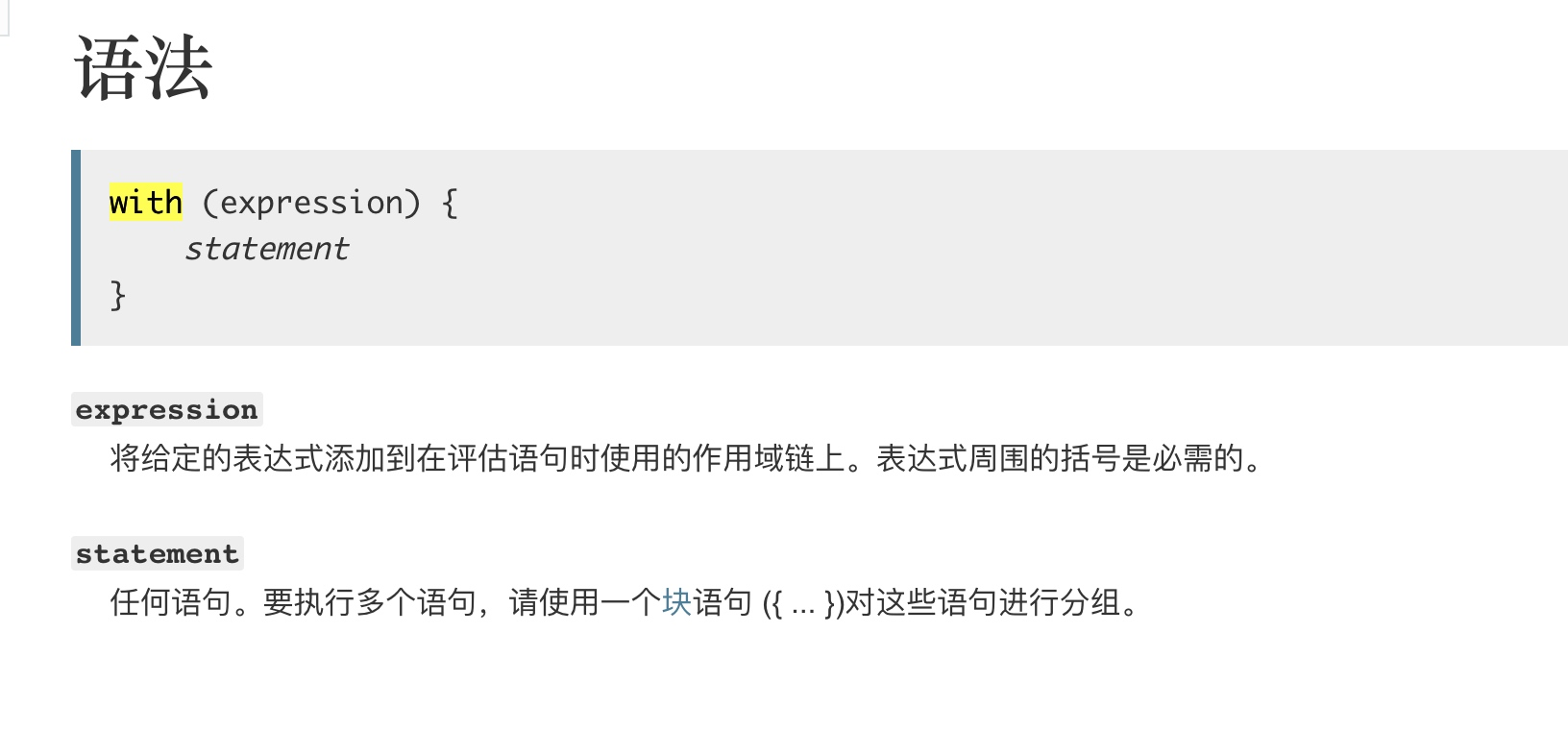`with`用法还是比较有趣的，它的产生方便了对象的属性调用，有了它就不需要重复引用对象本身。我们先来看一个正常的例子。

``````myobj = {
name : 'hpdoger',
sex : 'boy'
}

console.log(myobj.name)  //hpdoger
console.log(myobj.sex)  //boy

with(myobj){
console.log(name)  //hpdoger
console.log(sex) //boy
}``````

``````function foo(obj) {
with (obj) {
return __proto__
}
}

aa = {
name: 'boy'
}

``````function genevil(foo) {
with (foo) {
}
}
genevil(window)`/hpdoger/`;``````

``````flag = "flag{aaaa}";

function anonymous() {
with(par)return constructor
}

function par(a) {
console.log(123);
}

console.log(anonymous``)
console.log(anonymous`` `return flag` ``)``````

``````function getUrlParam(name) {
var reg = new RegExp("(^|&)" + name + "=([^&]*)(&|\$)");
var r = window.location.search.substr(1).match(reg);
if (r != null) return unescape(r); return null;
}

function init(Content,values='index.html'){
with (Content) {
title = 'XSS Demo';
page = values;
location = values;
}
}

let values = location.hash.slice(1);
let uid = getUrlParam("role");

title:'',
page:''
}

let ContentUser = {
title:'',
location:''
}

init(ContentUser,values);
}else{
}``````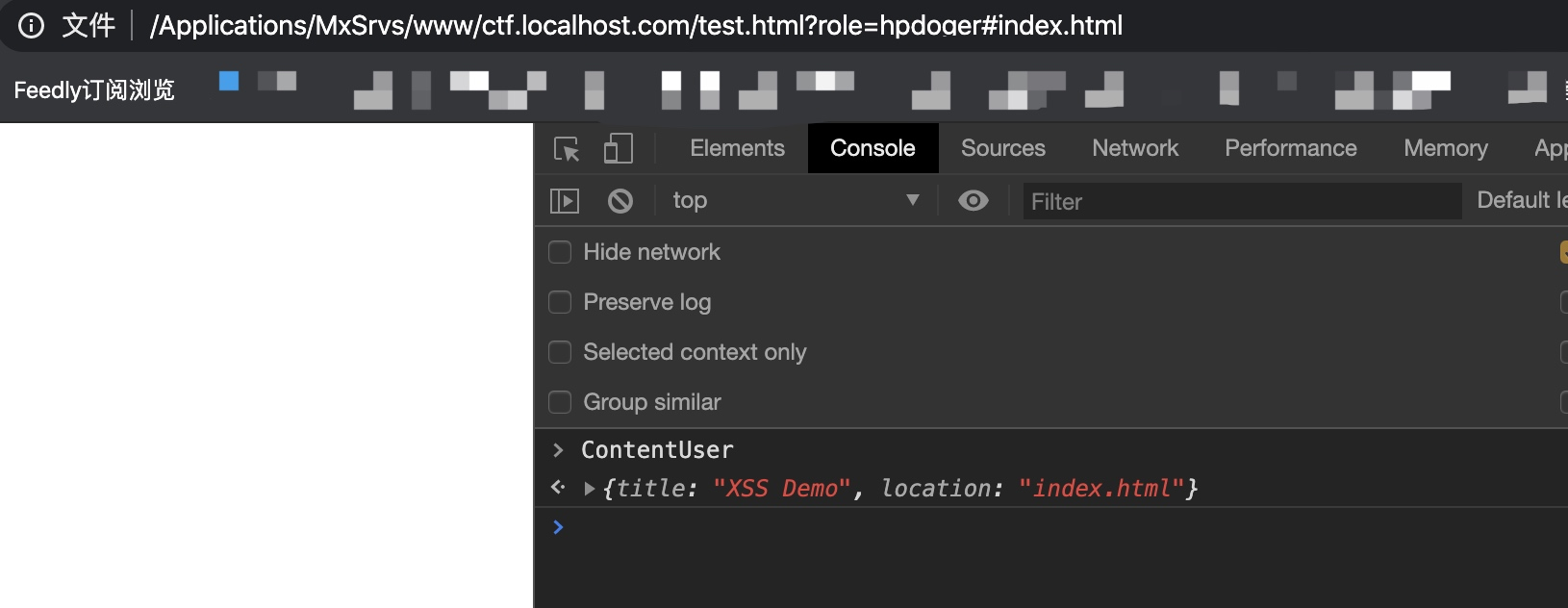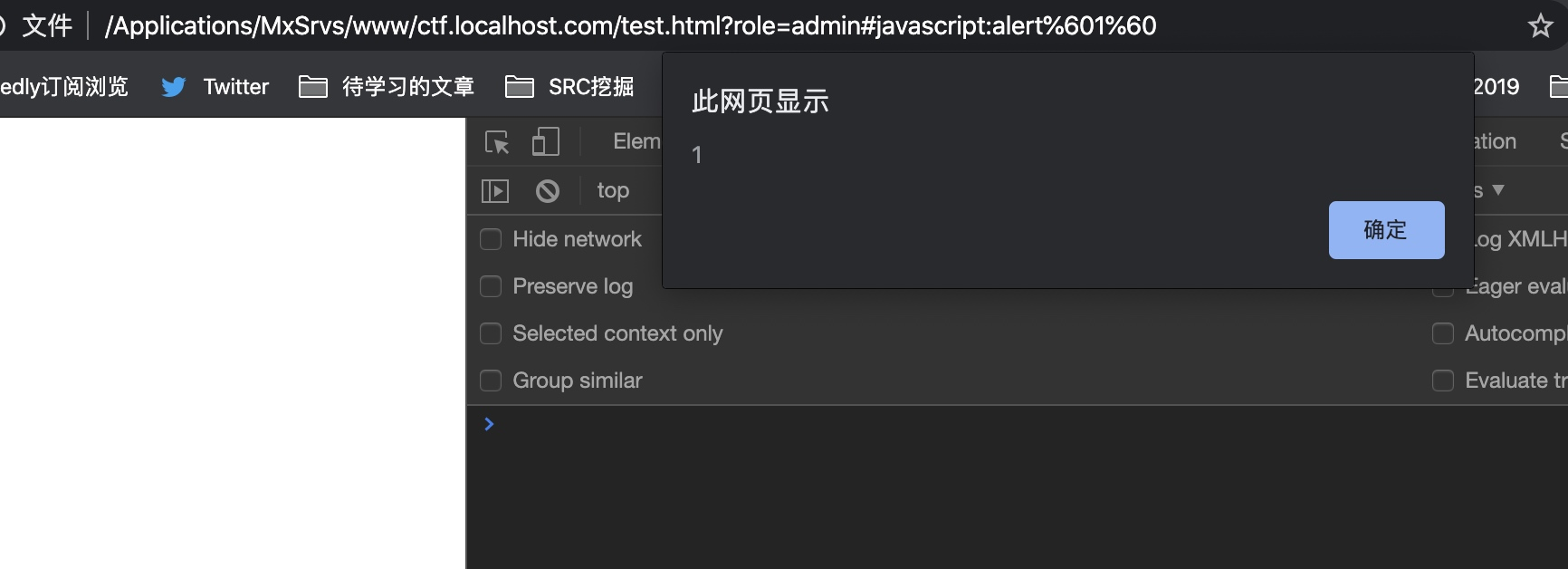## this的绑定

``````var myobj = {
getbar : function(){
console.log(this.bar);
},
bar: 1

};

var bar = 2;
var getbar = myobj.getbar;

myobj.getbar()//1
getbar(); //2``````# 最后

•